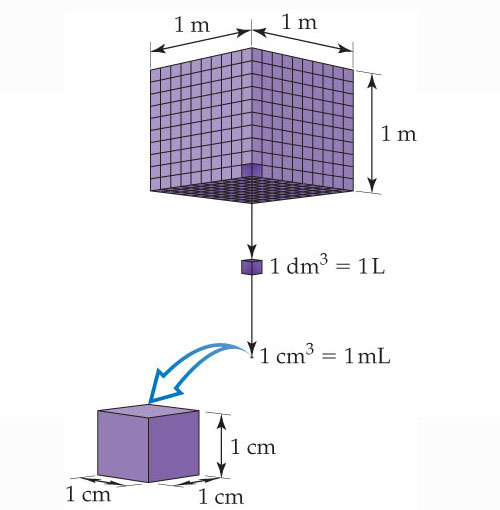# Problem: Volume relationships. The volume occupied by a cube 1 m on each edge is one cubic meter, 1 m3. Each cubic meter contains 1000 dm3. One liter is the same volume as one cubic decimeter, 1 L = 1 dm3. Each cubic decimeter contains 1000 cubic centimeters, 1 dm3 = 1000 cm3. One cubic centimeter equals one milliliter, 1 cm3 = 1 mL.How many 1-L bottles are required to contain 1 m3 of liquid?

###### FREE Expert Solution

We are asked how many 1-L bottles are required to contain 1 m3 of liquid?

This simply means that we need to convert the volume to liters.

84% (120 ratings)###### Problem DetailsVolume relationships. The volume occupied by a cube 1 m on each edge is one cubic meter, 1 m3. Each cubic meter contains 1000 dm3. One liter is the same volume as one cubic decimeter, 1 L = 1 dm3. Each cubic decimeter contains 1000 cubic centimeters, 1 dm3 = 1000 cm3. One cubic centimeter equals one milliliter, 1 cm3 = 1 mL.

How many 1-L bottles are required to contain 1 m3 of liquid?